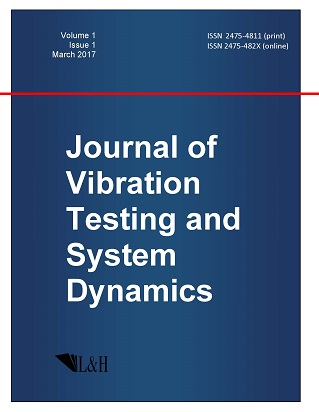ISSN: 2475-4811 (print)
ISSN: 2475-482X (online)
Journal of Vibration Testing and System Dynamics

C. Steve Suh (editor), Pawel Olejnik (editor),

Xianguo Tuo (editor)

Pawel Olejnik (editor)

Lodz University of Technology, Poland

Email: pawel.olejnik@p.lodz.pl

C. Steve Suh (editor)

Texas A&M University, USA

Email: ssuh@tamu.edu

Xiangguo Tuo (editor)

Sichuan University of Science and Engineering, China

Email: tuoxianguo@suse.edu.cn

On the 100th Anniversary of the Full Member of Russian Academy Of Sciences L.V. Ovsyannikov and the 80th Anniversary of His Follower, Professor N.H. Ibragimov

Journal of Vibration Testing and System Dynamics 5(3) (2021) 207--219 | DOI:10.5890/JVTSD.2021.09.001

Inna Emelyanova

Campbell, California, USA

Abstract

Two dates coincided in 2019: the centenary of the full member of Russian Academy of Sciences Lev Vasilievich Ovsyannikov (April 22, 1919 -- May 23, 2014) and the eightieth anniversary of his follower, professor Nail Hairullovich Ibragimov (January 18, 1939 -- November 04, 2018). Through the efforts of them and their numerous students and followers, Sophus Lie's theory of local transformation groups was revived and received promising development.

References

2.   Gaston, D.J. (2013), Lectures on general theory of surfaces and geometrical applications of the analysis of infinitely small. In 4 volumes: Fr. - M.; Izhevsk: in-t-computer study.
3.   Titov, V.M., Chupakhin, A.P., and Ovsiannikov, L.V. (1999), (To the 80th Birthday Anniversary), Applied mathematics and mechanics, 63(3), 355-359.
4.   Ovsyannikov, L.V. (1958), Groups and Invariant-Group Solutions of Differential Equations, Dokl. of the USSR Academy of Sciences, 118(3), 439-442.
5.   Negin, E.A., Goleusova, L.P., Kulichkov, G.D. et al. (1995), Soviet atomic project. (in Russian) - Nizhny Novgorod; Arzamas-16: Nizhny Novgorod Publishing House, - 296 c.
6.   Mathematical problems of liquid and gas mechanics (in Russian) // Science in Siberia. 2004. 16. P.5. (Scientific school of academician L.V. Ovsiannikov. To the 85th anniversary of his birth).
7.   Ovsyannikov, L.V. (1962), Group properties of the differential equations (in Russian). - Novosibirsk: Published in Siberian Branch of AS USSR - 238 c.
8.   Ovsyannikov, L.V. (1978), Group Analysis of the Differential Equations (in Russian). - Moscow: Nauka, - 399 c.
9.   Ovsyannikov, L.V. (1989), (To the seventieth anniversary of his birth), Applied mathematics and mechanics, 53(2), C.179--183.
10.   Ibragimov N.H. (2007), Practical course of the differential equations and mathematical modeling. Classical and new methods. Nonlinear mathematical models. Symmetry and principles of invariance (in Russian) /Translated by I.S. Emelyanova. Nizhni Novgorod: NNSU Publishing House, - 421 p.; 2nd ed., additional and corrected. - Moscow: Fizmatlit, 2012. - 332 c. (Ibragimov N. A Practical Course in Differential Equations and Mathematical Modelling: Classical and new methods, nonlinear mathematical models, symmetry and invariance principles, 3d ed. Blekinge Inst. Technology, Karlskrona, Sweden: ALGA publ., 2006. -370 p.).
11.   Ibragimov, N.H. (2006), Selected works. Vol. 1 Blekinge Institute of Technology, Karlskrona, Sweden: ALGA Publications, -280 p.
12.   Ibragimov N.H. (1983), Groups of transformations in mathematical physics (in Russian). - Moscow: Nauka, - 280 p.
13.   Ibragimov, N.H., Ed. (1994-1996), CRC Handbook of Lie group analysis of differential equations. Vol. 1--3. CRS Press Inc., Boca Raton.
14.   Archives of ALGA. (2004-2012), Blekinge Institute of Technology, Karlskrona, Sweden: ALGA Publications, Volumes 1-9.
15.   Emelyanova I.S. (2008), First nominee for the Lagrangian Prize" (2008) - mathematician N.H. Ibragimov (in Russian) // Scientists notes of the Volgo-Vyatka Branch of the International Slavic Academy. Issue 23. Nizhny Novgorod. C. 14--18.
16.   Baikov, V.A. and Gazizov, R.K. (2018), In memory of a scientist (in Russian) // Ufa mathematical journal, 10(4), 138--140.
17.   Gazizov, R.K. (2009), The 70$^{th}$ anniversary of Nail Hayrullovich Ibragimov (in Russian), Ufa mathematical journal, 1(3), C. 160--163.